IMO Shortlist 2003 problem G3

Kvaliteta:
Avg: 0,0
Težina:
Avg: 7,0
Let$ABC$ be a triangle, and$P$ a point in the interior of this triangle. Let$D$,$E$,$F$ be the feet of the perpendiculars from the point$P$ to the lines$BC$,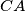$CA$,$AB$, respectively. Assume that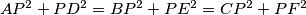$AP^{2}+PD^{2}=BP^{2}+PE^{2}=CP^{2}+PF^{2}$.

Furthermore, let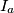$I_{a}$,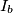$I_{b}$,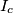$I_{c}$ be the excenters of triangle$ABC$. Show that the point$P$ is the circumcenter of triangle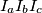$I_{a}I_{b}I_{c}$.
Izvor: Međunarodna matematička olimpijada, shortlist 2003